# Electrical Impedance: What is it? (Types & Examples)

## What is Electrical Impedance?

In electrical engineering, electrical impedance is the measure of the opposition that a circuit presents to a current when a voltage is applied. Impedance extends the concept of resistance to alternating current (AC) circuits. Impedance possesses both magnitude and phase, unlike resistance, which has only magnitude.

Unlike electrical resistance, electrical impedance’s opposition to current depends on the frequency of the circuit. Resistance can be thought of as impedance with a phase angle of zero.

A circuit where the current lags 90° (electrical) in respect of the applied voltage in a purely inductive circuit. A circuit where current leads 90° (electrical) in respect of the applied voltage in a purely capacitive circuit. A circuit where the current does not lag nor lead in respect to the applied voltage in a purely resistive circuit. When a circuit is driven with direct current (DC), there is no distinction between impedance and resistance.

In a practical circuit where both inductive reactance and capacitive reactance present along with resistance or either of capacitive or inductive reactance presents along with resistance, there will be leading or lagging effect on the current of the circuit depending on the value of reactance and resistance of the circuit.

In the AC circuit, the cumulative effect of reactance and resistance is termed as impedance. The impedance is normally denoted by English letter Z. The value of impedance is represented as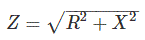Where R is the value of circuit resistance and X is the value of circuit reactance.
The angle between applied voltage and current is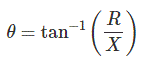The inductive reactance is taken as positive and capacitive reactance is taken as negative.

Impedance can be represented in complex form. This is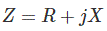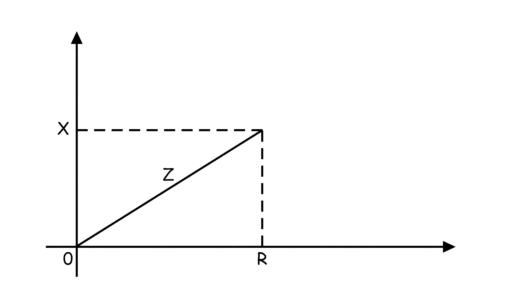The real part of a complex impedance is resistance and the imaginary part is reactance of the circuit.

Let us apply a sinusoidal voltage Vsinωt across a pure inductor of inductance L Henry.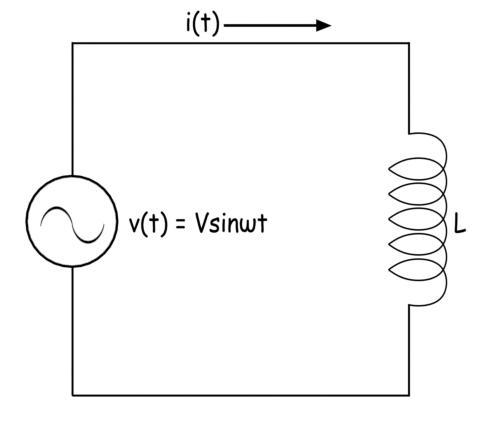The expression of current through the inductor isFrom the expression of the waveform of the current through the inductor it is clear that the current lags the applied voltage by 90° (electrical).

Now let us apply same sinusoidal voltage Vsinωt across a pure capacitor of capacitance C farad.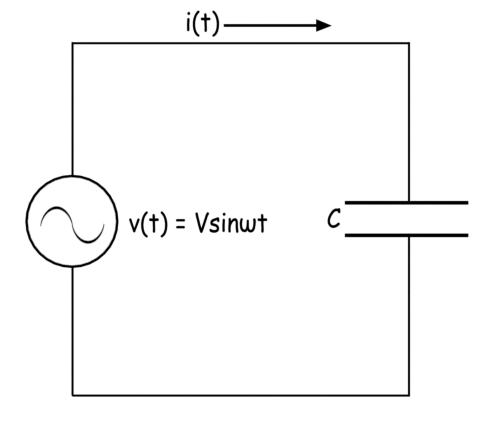The expression of current through the capacitor is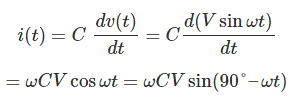From the expression of the waveform of the current through the capacitor it is clear that the current leads the applied voltage by 90°(electrical).

Now we will connect the same voltage source across a pure resistance of value R ohm.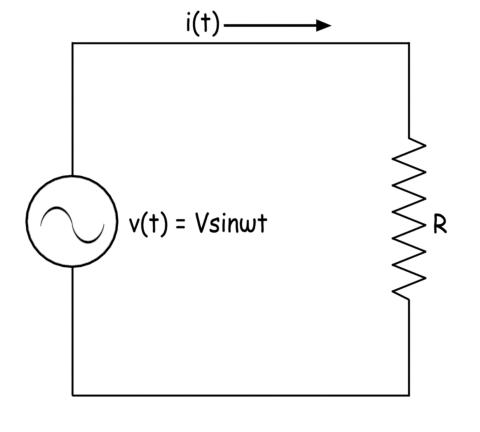Here the expression of current through the resistance would beFrom that expression, it can be concluded that the current has the same phase with the applied voltage.

## Impedance of a Series RL Circuit

Let us derive the expression of the impedance of a series RL circuit. Here resistance of value R and inductance of value L are connected in series. The value of reactance of the inductor is ωL. Hence the expression of impedance in complex form is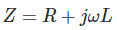The numerical value or mod value of the reactance is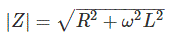## Impedance of a Series RC Circuit

Let us connect one resistance of value R ohm in series with a capacitor of capacitance C farad. The reactance of the capacitor is 1 / ωC. The resistance R and reactance of the capacitor are in series the expression of the impedance can be written as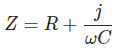The mod value of the impedance of the series RC circuit is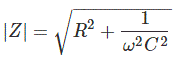## Impedance of a Parallel RL Circuit

Here the resistance and inductor are connected in parallel. Here reciprocal of the impedance of the circuit is the sum of the reciprocal of resistance and reciprocal of reactance.The expression of the impedance of the parallel RL circuit can be represented as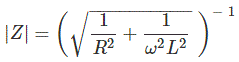## Impedance of a Parallel RC Circuit

Here as the capacitor and resistor are connected in parallel, the reciprocal of the impedance of the circuit is the sum of the reciprocal of resistance and reciprocal of the reactance of the capacitor.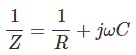Finally, we can write the expression of the impedance of the parallel RC circuit as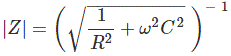## Impedance of a Series RLC Circuit

Here resistors, capacitors, and inductors are connected in series. The total reactance of the circuit is the sum of the reactance of inductors and capacitors. The reactance of the capacitors is taken as negative. The expression of impedance of a series RLC circuit is## Impedance of a Parallel RLC Circuit

Here resistor, capacitor, and inductor are connected in parallel. In the same way, the equivalent impedance of a parallel RLC circuit can be determined and the finally the expression of the impedance of a parallel RLC circuit is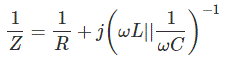## Polar Representation of Impedance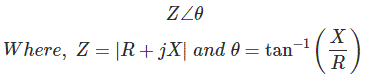Want To Learn Faster? 🎓
Get electrical articles delivered to your inbox every week.
No credit card required—it’s 100% free.